Question

# 1. Write the half‑reaction for the silver-silver chloride reference electrode. Include physical states. 2. Write the...

1. Write the half‑reaction for the silver-silver chloride reference electrode. Include physical states.

2. Write the half‑reaction for the saturated calomel reference electrode. Include physical states.

3. What is the potential of the cell:

silver–silver chloride electrode || saturated calomel electrode

given that the potential for the Ag∣∣AgCl electrode in a saturated KCl solution is +0.197 V and the potential for a saturated calomel electrode is +0.241 V?

We need at least 10 more requests to produce the answer.

0 / 10 have requested this problem solution

The more requests, the faster the answer.

All students who have requested the answer will be notified once they are available.

#### Earn Coins

Coins can be redeemed for fabulous gifts.

Similar Homework Help Questions
• ### Write the half-reaction for the silver-silver chloride reference electrode. Include physical states. half-reaction: Write the half-reaction...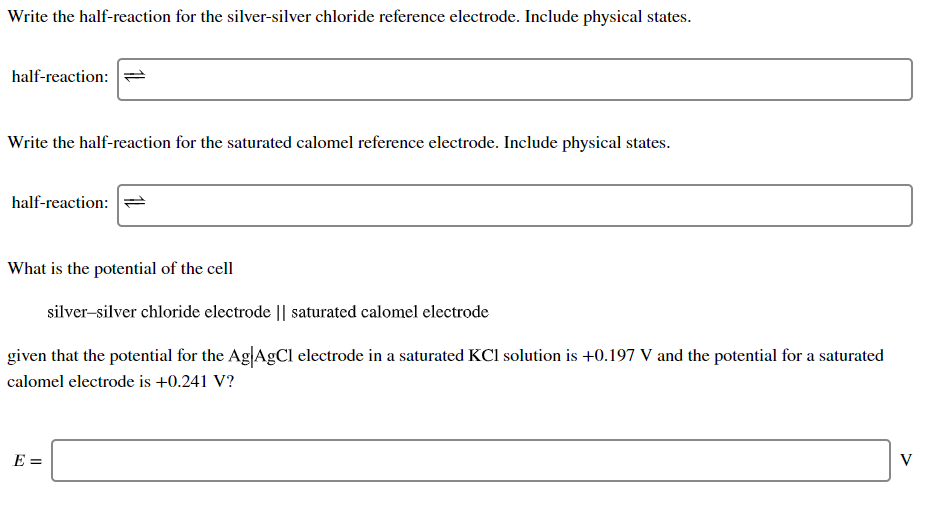Write the half-reaction for the silver-silver chloride reference electrode. Include physical states. half-reaction: Write the half-reaction for the saturated calomel reference electrode. Include physical states. half-reaction: What is the potential of the cell silver-silver chloride electrode || saturated calomel electrode given that the potential for the Ag AgCl electrode in a saturated KCl solution is +0.197 V and the potential for a saturated calomel electrode is +0.241 V? E=

• ### Write the half-reaction for the silver-silver chloride reference electrode. Include physical stat...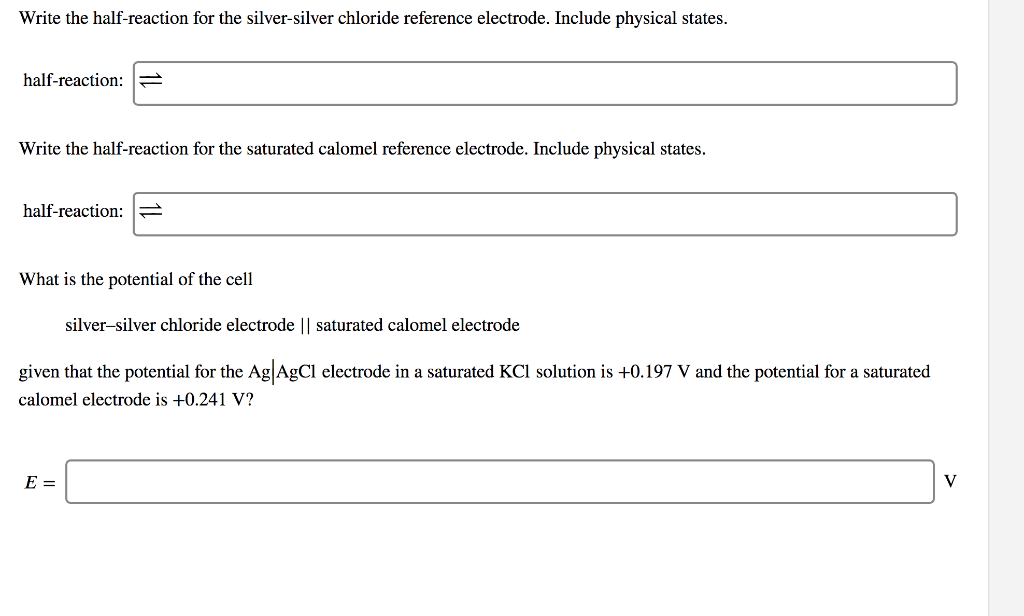Write the half-reaction for the silver-silver chloride reference electrode. Include physical states. half-reaction: - Write the half-reaction for the saturated calomel reference electrode. Include physical states half-reaction: What is the potential of the cell silver-silver chloride electrode || saturated calomel electrode given that the potential for the AglAgCI electrode in a saturated KCl solution is +0.197 V and the potential for a saturated calomel electrode is +0.241 V? Write the half-reaction for the silver-silver chloride reference electrode. Include physical states....

• ### Convert each of the potentials. The silver-silver chloride and calomel reference electrodes are saturated with KCl,...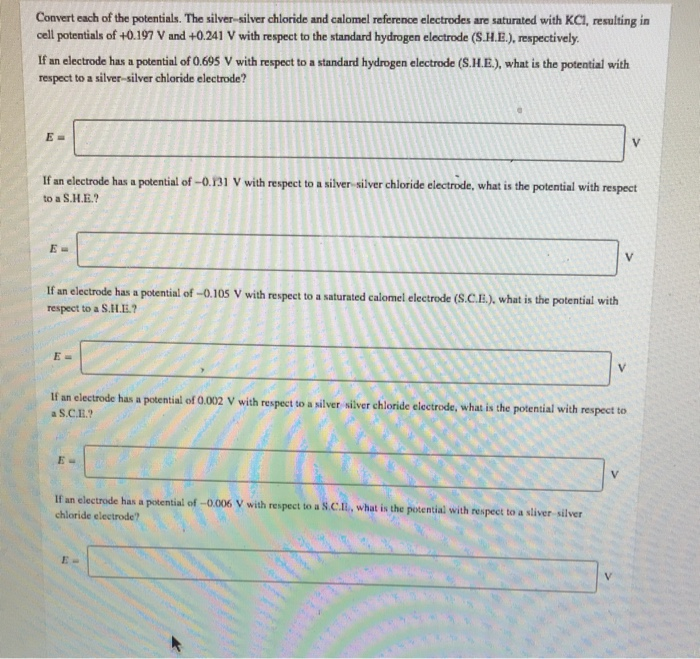Convert each of the potentials. The silver-silver chloride and calomel reference electrodes are saturated with KCl, resulting in cell potentials of +0.197 V and +0.241 V with respect to the standard hydrogen electrode (S.H.E.), respectively If an electrode has a potential of 0.695 V with respect to a standard hydrogen electrode (S.H.E.), what is the potential with respect to a silver-silver chloride electrode? E= V If an electrode has a potential of-0.131 V with respect to a silver-silver chloride electrode,...

• ### Convert each of the potentials. The silver-silver chloride and calomel reference electrodes are saturated with KCl,...

Convert each of the potentials. The silver-silver chloride and calomel reference electrodes are saturated with KCl, resulting in cell potentials of +0.197 V and +0.241 V with respect to the standard hydrogen electrode (S.H.E.), respectively. If an electrode has a potential of 0.496 V with respect to a standard hydrogen electrode (S.H.E.), what is the potential with respect to a silver–silver chloride electrode? If an electrode has a potential of −0.147 V with respect to a silver–silver chloride electrode, what...

• ### Convert each of the potentials. The silver–silver chloride and calomel reference electrodes are saturated with KCl,...

Convert each of the potentials. The silver–silver chloride and calomel reference electrodes are saturated with KCl, resulting in cell potentials of +0.197 V and +0.241 V with respect to the standard hydrogen electrode (S.H.E.), respectively. If an electrode has a potential of 0.686 V with respect to a standard hydrogen electrode (S.H.E.), what is the potential with respect to a silver–silver chloride electrode? E= If an electrode has a potential of −0.118 V with respect to a silver–silver chloride electrode,...

• ### i answered 0.58 but I got it wrong. can you help/solve? Question: A chemist measures the...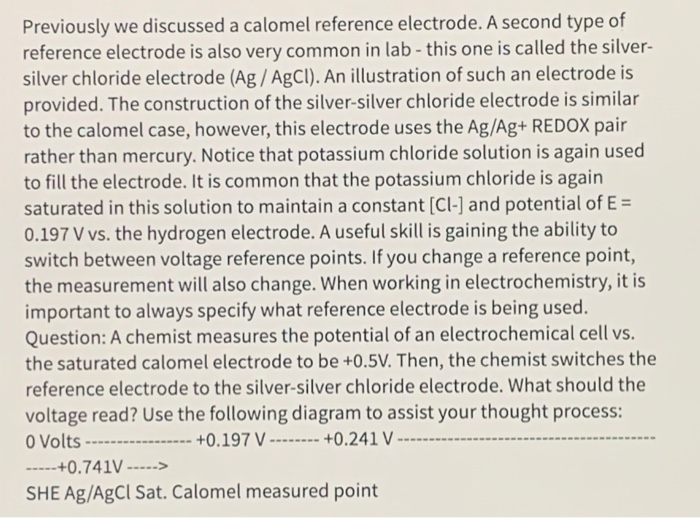i answered 0.58 but I got it wrong. can you help/solve? Question: A chemist measures the potential of an electrochemical cell vs. the saturated calomel electrode to be +0.5V. Then, the chemist switches the reference electrode to the silver-silver chloride electrode. What should the voltage read? Use the following diagram to assist your thought process: Previously we discussed a calomel reference electrode. A second type of reference electrode is also very common in lab - this one is called the...

• ### Calculate the cell potential (E) for the half-reaction of a silver-silver chloride reference electrode (E =...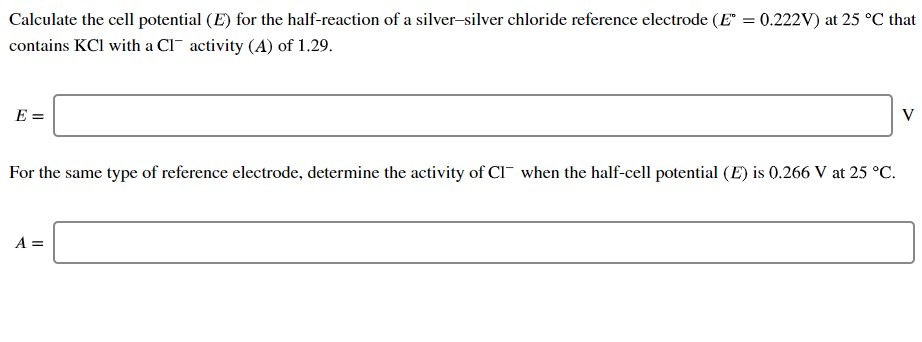Calculate the cell potential (E) for the half-reaction of a silver-silver chloride reference electrode (E = 0.222V) at 25 °C that contains KCl with a clºactivity (A) of 1.29. E = For the same type of reference electrode, determine the activity of C1 when the half-cell potential (E) is 0.266 V at 25 °C. A=

• ### Convert the following voltages Assume the silver-siIver chloride and calomel reference electrodes are saturated with KCl,...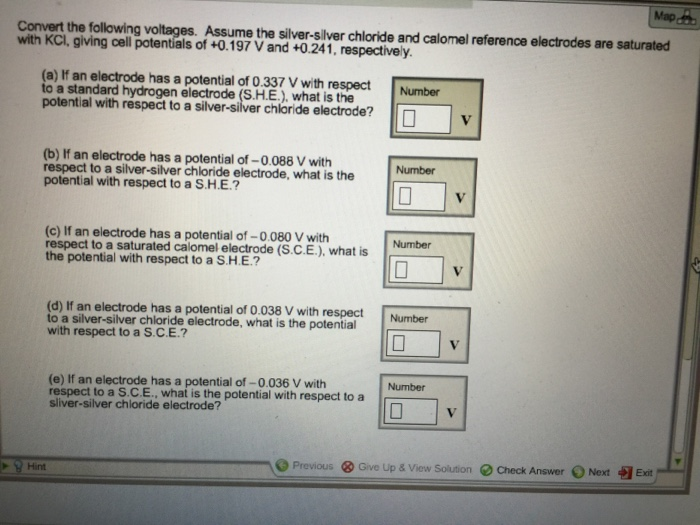Convert the following voltages Assume the silver-siIver chloride and calomel reference electrodes are saturated with KCl, giving cell potentials of +0.197 V and +0 241 respective y If an electrode has a potential of 0.337 V with respect to a standard hydrogen electrode (S H E ), what is the potential with respect to a silver-silver chloride electrode? If an electrode has a potential of -0 088 V with respect to a silver-silver chloride electrode, what is the potential with...

• ### draw a diagram like figure 15-6 to convert the following potentials. The Ag|AgCl and calomel reference...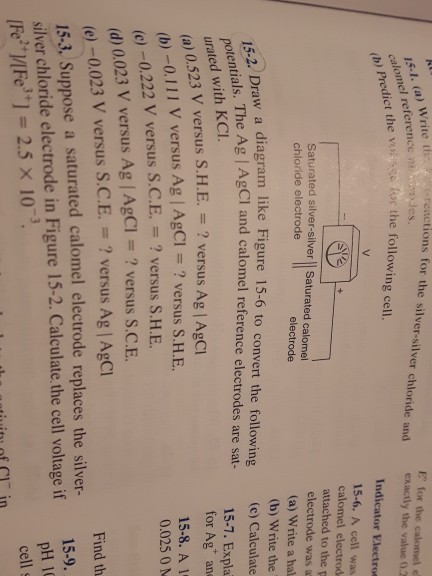draw a diagram like figure 15-6 to convert the following potentials. The Ag|AgCl and calomel reference elctrodes are saturated with KCl rcactions for the silver-silver chloride and exacly the vanue 15-1. (a) Write ts lomel val sse for the following cell. E for the calomel e (b) Predict the Predict t Indicator Electroe 15-6. A cell was calomel electrod attached to the electrode was a (a) Write a hal (b) Write the (c) Calculate Saturated silver-silver Saturated calomel chloride electrode...

• ### A cell consists of a gold wire and a saturated calomel electrode (S.C.E.) in a 0.170 M AuNO, solu...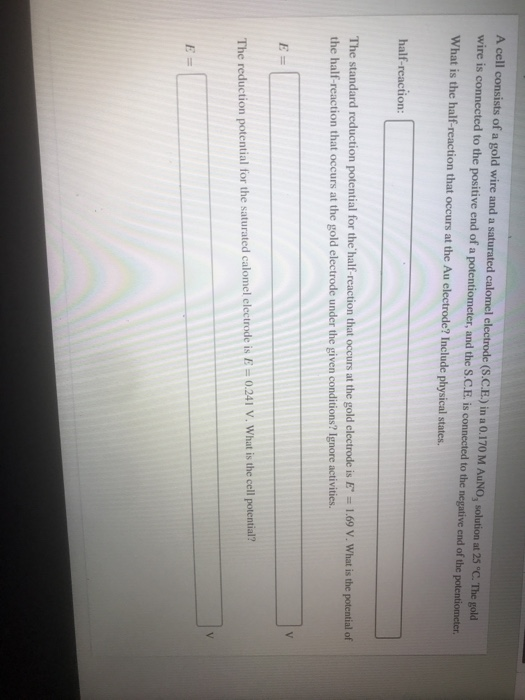A cell consists of a gold wire and a saturated calomel electrode (S.C.E.) in a 0.170 M AuNO, solution at 25 °C. The gold wire is connected to the positive end of a potentiometer, and the S.C.E. is connected to the negative end of the potentiometer. What is the half-reaction that occurs at the Au electrode? Include physical states. half-reaction: The standard reduction potential for the half reaction that occurs at the gold clectrode is E 1.69 V. What is...